# EE204: Business Management for Electrical Engineers and Computer Scientists

## The Rule of 72

In finance, the rule of 72, the rule of 70 and the rule of 69.3 all refer to essentially the same method for estimating doubling times for exponential growth or halving times for exponential decay. If you divide the number given by the expected growth rate, expressed as a percentage, the answer is approximately the number of periods to double the original quantity. For instance, if you were to invest \$100 at 9% per annum, then your investment would be worth \$200 after 8.0432 years, using an exact calculation. The rule of 72 gives 72/9 = 8 years, which is close to the exact answer. See time value of money. The same applies to exponential decay. Thus to determine the time it takes for the value of money to halve due to a given inflation rate, financiers simply take the current inflation rate (approx 3.5% until recently) and divide 70 by that number. Thus, 70/3.5 would give 20. This means that at 3.5% inflation it should take 20 years for the value of a dollar to halve.

The number 72 is a better approximation for annual interest compounding at typical rates. For continuous compounding ln (2), which is about 69.3%, will give accurate results for any rate. Daily compounding is close enough to continuous compounding for most purposes, so 69.3 or 70 should be used. The value 72 is also a convenient choice since it has so many small divisors: 2, 3, 4, 6, 8, 9, and 12.

### Derivation

For periodic compounding future value is given bywhere PV is the present value, t is the number of time periods, and r stands for the discount rate per time period.

Now, suppose that the money has doubled, then FV = 2PV. Substituting this in the above formula and cancelling the factor PV on both side yields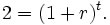This equation is easily solved for t:If r is small, then ln(1+r) approximately equals r (this is the first term in the Taylor series). Together with the approximation ln 2 ≈ 0.693147, this gives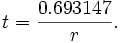So, for very small rates, 69.3 would be more accurate than 72. For higher rates, a bigger numerator would be better (e.g. for 20%, using 76 to get 3.8 years would be only about 0.002 off, where using to 72 get 3.6 would be about 2.002 off). 72 is a reasonable approximation across this range and is easily divisible by many numbers. The rule of 72 is only an approximation that is accurate for a range of interest rate (from 6% to 10%). Outside that range the error will vary from 2.4% to 14.0%. It turns out that for every three percentage points away from 8% the value 72 could be adjusted by 1.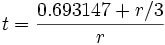(approximately)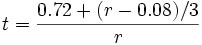(approximately)

### Continuous compounding

For continuous compounding the derivation is simpler: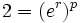impliesor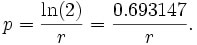Using 100r to get percentages and taking 70 as a close enough approximation to 69.3147: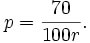For a discussion of the approximation error you get from the Rule of 72 see http://mathworld.wolfram.com/Ruleof72.html